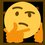# Golden Ratio Version 2

\begin {aligned} \frac{a}{b}&=\frac{a+b}{a}\\ a^2&=ba+b^2\\ a^2-ba&=b^2\\ a^2-2\left(\frac{b}{2}\right)a+\left(\frac{b}{2}\right)^2&=b^2+\left(\frac{b}{2}\right)^2\\ \left(a-\frac{b}{2}\right)^2&=b^2+\frac{b^2}{4}\\ a-\frac{b}{2}&=±\sqrt{\frac{5b^2}{4}}\\ a&=\frac{b}{2}±\frac{\sqrt5b}{2}\\ a&=b\left(\frac{1±\sqrt5}{2}\right) \end {aligned}

\begin{aligned} \phi&\overset{\text{def}}{=}\frac{a}{b}\\ &=\frac{b\left(\frac{1±\sqrt5}{2}\right)}{b}\\ &=\boxed{\frac{1±\sqrt5}{2}} \end{aligned}Note by Gandoff Tan
1 year, 8 months ago

This discussion board is a place to discuss our Daily Challenges and the math and science related to those challenges. Explanations are more than just a solution — they should explain the steps and thinking strategies that you used to obtain the solution. Comments should further the discussion of math and science.

When posting on Brilliant:

• Use the emojis to react to an explanation, whether you're congratulating a job well done , or just really confused .
• Ask specific questions about the challenge or the steps in somebody's explanation. Well-posed questions can add a lot to the discussion, but posting "I don't understand!" doesn't help anyone.
• Try to contribute something new to the discussion, whether it is an extension, generalization or other idea related to the challenge.

MarkdownAppears as
*italics* or _italics_ italics
**bold** or __bold__ bold
- bulleted- list
• bulleted
• list
1. numbered2. list
1. numbered
2. list
Note: you must add a full line of space before and after lists for them to show up correctly
paragraph 1paragraph 2

paragraph 1

paragraph 2

[example link](https://brilliant.org)example link
> This is a quote
This is a quote
    # I indented these lines
# 4 spaces, and now they show
# up as a code block.

print "hello world"
# I indented these lines
# 4 spaces, and now they show
# up as a code block.

print "hello world"
MathAppears as
Remember to wrap math in $$ ... $$ or $ ... $ to ensure proper formatting.
2 \times 3 $2 \times 3$
2^{34} $2^{34}$
a_{i-1} $a_{i-1}$
\frac{2}{3} $\frac{2}{3}$
\sqrt{2} $\sqrt{2}$
\sum_{i=1}^3 $\sum_{i=1}^3$
\sin \theta $\sin \theta$
\boxed{123} $\boxed{123}$